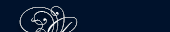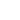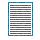НОВОСТИ   БИБЛИОТЕКА   ЭНЦИКЛОПЕДИЯ   КАРТА САЙТА   ССЫЛКИ   О САЙТЕFamily Ages. The father and the mother were both of the same age, thirty-six years, and the three children were triplets (тройней) of six years of age.

Three Curious Problems.

1. Farmer Jones is carrying empty bags.

2. Jill's grandfather was born in 1881.

3. 18 spokes.

The Bag of Nuts. The five bags contained 27, 25, 18, 16, 14 nuts. You can find how many nuts are in each bag if you subtract the two pairs together from 100. So, 100 - (52 + 30) = 18, the third bag.

An Age Problem. The man is sixty years old.

Find the Numbers. The two numbers are 11 and 1.1.

The Three Boys.

 Alec can do the work in 14 34 days; 49
 Bill - in 17 23 days; 41
 John - in 23 7 days. 31

Six Apples in a Bag. The sixth boy received his apple in the bag.

Hundreds and Hundreds.

1) 1 + 2 + 3 + 4 + 5 + 6 + 7 + (8 × 9) = 100.

 2) 8 × 8 = 1 8 8

3) (5 + 5)·(5 + 5)=100.

Strange Figures. Write, the following four numbers composed of five odd figures 11, 1, 1, 1. After addition you will have 14.

Here Are the Riddles

1. Place 3 pieces in one cup and 7 in another, and then place one of these in the third cup.

2. Give one of the boys the box with an orange in it.

3. 3 ducks.

4. XIX. Take 1 away and have XX.

5. Neither, but G and 7 are 13 and 6 and 7 is 13 are both correct.ПОИСК: